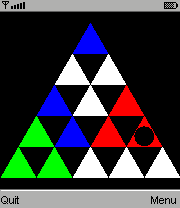-=+=- -=+=- -=+=- -=+=- -=+=- -=+=- -=+=- -=+=- -=+=- -=+=- -=+=- -=+=- -=+=- -=+=- -=+=- -=+=- -=+=- -=+=- -=+=- -=+=- -=+=- -=+=- -=+=- -=+=- -=+=- -=+=- -=+=- -=+=- -=+=- -=+=- (c) WidthPadding Industries 1987 0|60|0 -=+=- -=+=- -=+=- -=+=- -=+=- -=+=- -=+=- -=+=- -=+=- -=+=- -=+=- -=+=- -=+=- -=+=- -=+=- -=+=- -=+=- -=+=- -=+=- -=+=- -=+=- -=+=- -=+=- -=+=- -=+=- -=+=- -=+=- -=+=- -=+=- -=+=-
SoCoder -> Showcase Home -> Puzzle

PioCreated : 20 March 2008
Edited : 21 April 2009
Language : D

### Ruben's Pyramid

actually a triangle; shuffle then solve

jar file
ScreenshotsThis game is for mobile phone. It is a quick conversion of Javascript minigame included in one of my previous browser games.

The object is to shuffle it and solve it in a fashion somewhat similar to Rubik's cube. Clicking a triangle mirrors others in 3 directions (experiment to see actually how).

Controls:
 0 hold to shuffle *,# decrease/increase number of triangles arrows or 2468 move pointer select button or 5 click on triangle

Ruben is some bioenergist my friend made a movie about. He built a pyramid in his backyard, charging people for spending time underneath it, "filling themselves with cosmic energy". Some people claim similar experiences after playing this game.

Rough and fast version (on screenshot): jad, jar
Smooth and slow version (for faster phones): jad, jar
Touch screen smooth version (for fantastic phones): jad, jar
Source (all 3 versions, called quick, smooth and touch), coded in MIDlet Pascal 2
Homepage with preview

## Latest Update

0.9.2: now with 30% less flicker!
0.9.3: optional antialiased version (only for fast phones)
20 Apr 2009: touch screen version, and homepage with game embedded here